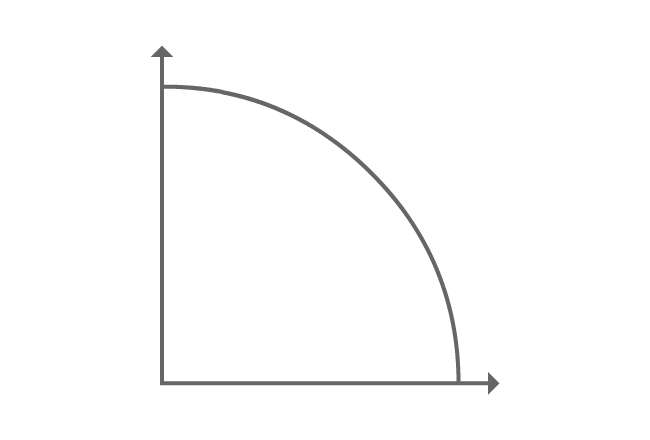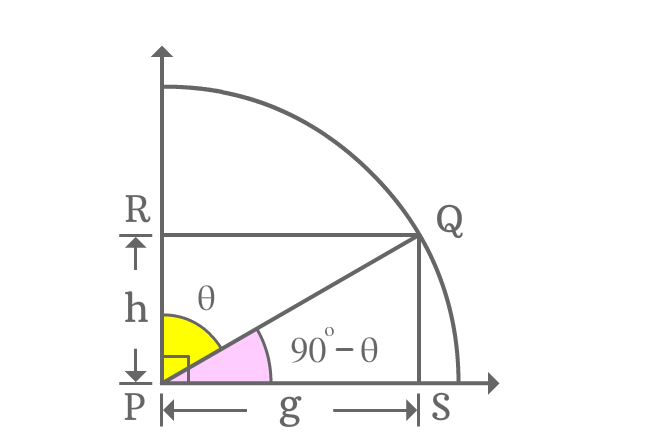# Cofunction identity of sec function

## Formula

Sexagesimal System
$\sec{(90^\circ-\theta)} \,=\, \csc{\theta} \,\,$ (or) $\,\, \operatorname{cosec}{\theta}$
$\sec{\Big(\dfrac{\pi}{2}-x\Big)} \,=\, \csc{x} \,\,$ (or) $\,\, \operatorname{cosec}{x}$

In cofunction identity, theta ($\theta$) is used to represent angle in degrees and $x$ is used to represent angle in radians.

Secant function contains an allied angle, which belongs to first quadrant. So, this trigonometric formula is called as first quadrant’s allied angle identity of sec function. The angles of both secant and cosecant functions are complementary angles in first quadrant. Therefore, this trigonometric identity is frequently called as cofunction identity of secant function.

### ProofIn first quadrant, a line segment is rotated to an angle and it is represented as $\overline{PQ}$.

A perpendicular line is drawn to horizontal ray and also another perpendicular line is drawn to vertical line from point $Q$. The two perpendicular lines are intersected the horizontal and vertical rays at points $S$ and $R$ respectively.

Thus, it formed two right angled triangles geometrically and they are known as $\Delta QPS$ and $\Delta QPR$.

Assume $\angle QPR = \theta$, then $\angle QPS = 90^\circ-\theta$.

If $PS = g$, then $RQ = g$ and take $RP = h$, then $QS = h$ mainly due to parallelism and equality of the line segments.

#### Express sec of allied angleThe angle of $\Delta QPS$ is an allied angle and it is $90^\circ-\theta$, which belongs to first quadrant. Write secant of allied angle of first quadrant as a ratio in terms of lengths of the corresponding sides.

$\sec{(90^\circ-\theta)} = \dfrac{PQ}{PS}$

The length of adjacent side is known but the length of hypotenuse is unknown. However, the length of opposite side is known. So, use Pythagorean Theorem to determine the length of the hypotenuse.

$\implies \sec{(90^\circ-\theta)} = \dfrac{\sqrt{g^2+h^2}}{g}$

#### Find equivalent value of the fractionSecant of allied angle of first quadrant is expressed as a ratio of lengths of the sides. Now, try to write the fraction in the form a trigonometric function and it is possible when $\Delta QPR$ is considered.

$\dfrac{\sqrt{g^2+h^2}}{g} = \dfrac{PQ}{RQ}$

The ratio of lengths of $\overline{PQ}$ to $\overline{RQ}$ represents cosecant and the angle of the triangle $\Delta QPR$ is theta. The value of the ratio of the sides is cosecant of angle theta.

$\implies \dfrac{\sqrt{g^2+h^2}}{g} = \csc{\theta}$

#### Cofunction identity of sec function

The above two mathematical steps derived geometrically that

$\sec{(90^\circ-\theta)}$ $=$ $\dfrac{\sqrt{g^2+h^2}}{g}$ $=$ $\csc{\theta}$

$\therefore \,\,\,\,\,\, \sec{(90^\circ-\theta)} \,=\, \csc{\theta}$

It is also written as follows in trigonometric mathematics.

$\therefore \,\,\,\,\,\, \sec{(90^\circ-\theta)} \,=\, \operatorname{cosec}{\theta}$

In first quadrant, it has proved that the value of secant of allied angle of first quadrant is equal to cosecant of angle. So, this trigonometric property is known as first quadrant’s allied angle identity of sec function.

Similarly, the angles of both secant and cosecant functions are complementary angles. So, the two functions are cofunctions. Therefore, it is called as cofunction identity of sec function.

A best free mathematics education website that helps students, teachers and researchers.

###### Maths Topics

Learn each topic of the mathematics easily with understandable proofs and visual animation graphics.

###### Maths Problems

A math help place with list of solved problems with answers and worksheets on every concept for your practice.

Learn solutions

###### Subscribe us

You can get the latest updates from us by following to our official page of Math Doubts in one of your favourite social media sites.